Deutsche VersionConversion
Voltage V to Voltage level Lu (dBu) and LV (dBV)
Voltage level Lu to LV and Voltage V Voltage level LV to Lu and Voltage V

Decibel  (dB)  level  conversion to volt
Level in dBu to dBV, or volts (rms), and dBV to dBu, or volts (rms)
volts (rms) to level in dBu and dBV       p-p = peak to peak

Conversion of sound units SPL (levels)
Conversion of factor, ratio, or gain to a level value (gain decibels dB)

 Level Lu dBu | Level LV dBV | Voltage V volts (RMS) | | ↓ | ↓ | ↓ | | Level LV dBV | Level Lu dBu | Level Lu dBu Voltage V volts (RMS) | Voltage V volts (RMS) | Level LV dBV | | volts (p-p) | volts (p-p) |

Calculator used with kind permission of DoctorProAudio.com
Fill out a gray box above and click the respective 'calculate' bar under it. p-p = peak to peak.
The reference voltage for 0 dBu is 0.775 volt (0.77459667 V) and for 0 dBV it is exactly 1.0 volt.
Scroll down to find the formulas for voltage and power and the calculation of the absolute level.

 The origin of the index of dBu comes from "u = unloaded" and dBV comes from "V = 1 volt". Some say: The "u" in dBu implies that the load impedance is unspecified, unterminated, and is likely to be high.   What is dBu? A logarithmic voltage ratio with a reference voltage of V0 = 0.7746 volt ≡ 0 dBu What is dBV? A logarithmic voltage ratio with a reference voltage of V0 = 1.0000 volt ≡ 0 dBV The home recording level (consumer audio) of −10 dBV means 0.3162 volts, that is −7.78 dBu.  The studio recording level (pro audio) of +4 dBu means a voltage of 1.228 volts. The maximum undistorted level of audio amplifiers is +18 dBu. In USA it is +24 dBu. Domestic gear with a −10 dBV level is usually unbalanced. Studio gear with a +4 dBu level is always balanced. 0 VU = +4 dBu.

Scale: Level in dBu and dBV in comparison to the voltage in V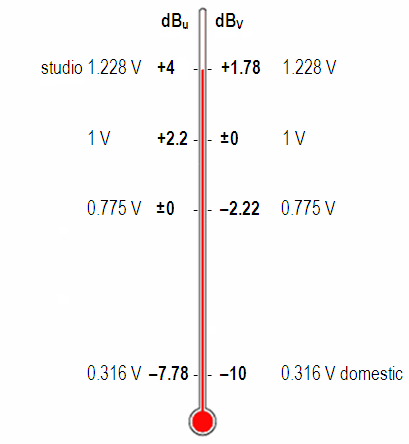Level   dBu Voltage volt Level   dBV Studio level international +4   ● 1.228 +1.78 Standard level 1 Volt +2.22 1 0 ref. Standard level 0.775 Volt 0 ref. 0.775 −2.22 Domestic level −7.78 0.316 −10   ●

 The level difference between +4 dBu studio level and −10 dBV consumer level is Δ L = 11.78 dB (12 dB).   The level difference between dBu level and dBV level is Δ L = 2.2 dB. 0 dBV equals 2.2 dBu or 0 dBu equals −2.2 dBV.   The conversion from level L(dBu) to voltage (volt) is V = 0.775 × 10(L/20). The conversion from voltage V (volt) to level (dBu) is L = 20 × log (V/0.775).

 All field quantities, like voltage or sound pressure are always true RMS values, if not otherwise stated.   In mathematics, the root mean square (abbreviated RMS or rms), also known as the quadratic mean, is a statistical measure of the magnitude of a varying quantity.

 For sinusodial voltages or currents with ohm's loads calculations can made easier with RMS = amplitude / √2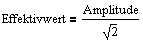Level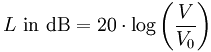Voltage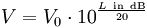Note - Comparing dBSPL and dBA: There is no conversion formula for measured dBA values to sound pressure level dBSPL or vice versa. Also you cannot convert "dBA to volts" and vice versa. Conversion is only possible for measuring one single frequency.

 Pro audio equipment often lists an A-weighted noise spec – not because it correlates well with our hearing – but because it can "hide" nasty hum components that make for bad noise specs.   Words to bright minds: Always wonder what a manufacturer  is hiding when they use A-weighting. *)

 We don't use the dBm in audio engineering. That belongs to power, we don't need here.   If you really need dBm with "m = 1 milliwatt or one thousandth (10−3) of a watt", go to: The dBm calculator and power matching or impedance matchingExplanation: What is "dBFS"? (Digital Audio)

dBFS - Digital recording level

 Analog levels and digital levels are different realms.

An often posted question: "Please, can you help me convert from dBFS to dBu".
Never express analog signal levels in terms of dBFS.
Follow this and you will not confuse anyone.

 There is no decibel to dBFS converter   Notice - Comparing dBu and dBFS: There is really no fixed world standard like e.g. −20 dBFS = +4 dBu = 0dBVU. The digital peak scale is not equivalent to the analog RMS scale.    You can never match dBFS and dBu.

 dBu is volts - you measure it with a volt meter.  Analog audio: positive and negative voltage.   dBFS is in contrast a binary number. Digital audio: zeroes and ones.

 There is no such thing as peak volts dBu *)   It is incorrect to state peak voltage levels in dBu.

*) http://www.rane.com/note169.html
*) http://www.rane.com/pdf/ranenotes/No_Such_Thing_as_Peak_Volts_dBu.pdf

dBFS must have a minus sign at the beginning. There is not something like +6 dBFS.

 Note: The guidelines of the EBU to set the maximum gain to −9 dB dBFS should not apply if not working for the European Broadcasting Union. Whose maximum levels of digital CD masters are −9dBFS, should not be surprised if the CDs are not loud enough. 9 dB up to the top are left free with really no use.

 More readable papers of the topic "Loudness and Level":   10 things you need to know about ... EBU R 128 - the EBU loudness recommendation   Florian Camerer: Loudness On the way to nirvana - audio levelling with EBU R 128

 LUFS = Loudness Units relative to Full ScaleThe formulas for voltage and power
and the calculation of the absolute level

 To use the calculator, simply enter a value. The calculator works in both directions of the ↔ sign.

 Voltage V  volts ↔ Voltage level LV dBV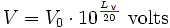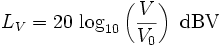Reference voltage V0 = 1 Volt ≡ 0 dBV
 Voltage V (audio)  volts ↔ Voltage level LU (audio)  dBu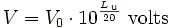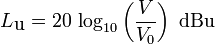Reference voltage V0 = 0.7746 Volt ≡ 0 dBu
 Electric power P  watts ↔ Electric power level LP dB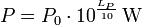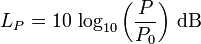Reference electric power P0 = 1 W ≡ 0 dB

dBm indicates that the reference power is P0 = 1 milliwatt = 0.001 watt ≡ 0 dB

 Electric power P (telephone)  watts ↔ Electric power level LP dBmReference power P0 = 1 milliwatt (mW) = 0.001 W ≡ 0 dBm

The parameters of the mains or "power" sine wave form are summarized at the table below:

 Average voltage RMS voltage (VRMS) Peak voltage (Vp) = (Û) Peak-to-peak voltage (Vpp) 0 volts 117 volts = VRMS = ~V 165 volts = √2×VRMS = 0,5 × Vpp 330 volts = 2×√2×VRMS = 2 × Vp 0 volts 230 volts = VRMS = ~V 325 volts = √2×VRMS = 0,5 × Vpp 650 volts = 2×√2×VRMS = 2 × Vp

 The value VRMS of an alternating voltage V (t) = V0 × f(t)is defined so that the effective DC power corresponds VRMS2 / R = VRMS × IRMS to an ohmic resistance of the middle resistive power of this AC voltage to the same resistance.

 Enter one known voltage: VRMS = Volt Vp      = Volt Vpp    = Volt Do not reenter an answer!The crest factor means the ratio of the peak voltage to the RMS voltage.
If you need to calculate an attenuator (attenuation calculation) you calculate a voltage divider.

Voltage conversions

 Voltage VRMS = ~V Vp Vpp Average voltage RMS VRMS  = − 0.7071 × Vp 0.3535 × Vpp Peak voltage Vp   = 1.414 × VRMS − 0.5000 × Vpp Peak-to-peak voltage   Vpp = 2.828 × VRMS 2.000 × Vp −

Different voltage levels

 Level Level L in dB Voltage RMS Voltage peak-to-peak European studio level - ARD broadcast level +6 dBu 1.55 V 4.38 V International studio level - USA +4 dBu 1.228 V 3.47 V Domestic recording (Consumer units) −10 dBV 0.3162 V ≡ −7.78 dBu 0.894 V Sound pressure level (auditory threshold) 0 dB 2×10−5 Pa ≡ 0 dBSPL 5.66×10−5 Pa Reference studio level re 0.775 volt 0 dBu 0.7746 V 2.19 V Reference studio level re 1 volt 0 dBV 1.0000 V 2.828 V

International reference values

 Physical unit Level unit Reference value Note Voltage V0 = 0.775 V ≡ 0 dBu Audio engineering, no impedance reference! Voltage V0 = 1 V ≡ 0 dBV Audio engineering, USA Voltage V0 = 1×10−6 V HF receiver and amplifier technology Voltage V0 = 0.224 V HF technology - Reference 1 mW at Z = 50 Ω Voltage V = 1.228 V Studio level +4 dBu, USA - Reference 0.7746 V Voltage V = 1.55 V Studio level +6 dBu, ARD - Reference 0.7746 V Voltage V = 0.3162 V Home recording level −10 dBV - Reference 1.0 V ≡ −7.78 dBu Sound pressure p0 = 2×10−5 Pa ≡ 0 dB Sound Pressure Level SPL, auditory threshold (Sound field size) Sound particle velocity v0 = 5×10−8 m/s ≡ 0 dB Sound intensity I0 = 1×10−12 W/m2 ≡ 0 dB Threshold of pain at 1 W/m2 (Sound energy size) Power P0 = 1 W ≡ 0 dBW The reference impedance must always be told Power P0 = 1 mW ≡ 0 dBm Z = 600 Ω (telephones) or Z = 50 Ω (antennas) Electric field strength E0 = 1×10−6 V/m

 Decibels (dB) Calculator   Decibels are defined as ten times the log of a power ratio. Decibels convert multiplication and division calculations into simple addition and subtraction operations.    This calculator converts between decibels, voltage gain (or current), and power gain. Just fill in one field and the calculator will convert the other two fields.   Equations: Level in dB:   L = 20 × log (V1/V2) = 10 × log (P1/P2)

 Decibels (dB) Voltage Gain Power Gain
 The dBm is a logarithmic measure of power compared to 1 mW,  that means it is power dependent. It can be converted to a voltage, if the load impedance is known. Typically the impedance (load) is 600 ohms.   Equation: Level in dBm:   LP = 10 × log (P / 0.001)

 Impedance = ohms dBm Power (watts) Voltage RMS Vp of sinusoid
 Simple rule of thumb: When working with power: 3 dB is twice, 10 dB is 10 times. When working with voltage or current: 6 dB is twice, 20 dB is 10 times.

 Why is the bandwidth and the cutoff frequency found at the level of "−3 dB"? Why we always take 3 dB down gain of a filter? Full width at half maximum (FWHM). Answer: That is the point where the energy (power) is fallen to the value ½ or 0.5 = 50 percent of the initial power as energy quantity, that is equivalent to (−)3 dB = 10×log(0.5). A (−)3 dB power drop is a decrease of 50 % to the value of 50%. There the voltage is fallen to the value of √(½) or 0.7071 = 70.71 percent of the initial voltage as field quantity equivalent to (−)3 dB = 20×log(0.7071). A (−)3 dB voltage drop is a decrease of 29.29 % to the value of 70.71 %.

 (−)3 dB implies ½ the electric power and since the power is proportional to the square of voltage, the value will be 0.7071 or 70.71 % of the passband voltage. √½ = 1/√2 = √0,5 = 0,7071.   P ~ V2, that is 0,5 ~ 0,70712.

 Sound engineers and sound designers ("ear people") mostly use the usual (sound) field quantity. That'swhy they say: The cutoff frequency of a device (microphone, amplifier, loudspeaker) is the frequency at which the output voltage level is decreased to a value of (−)3 dB below the input voltage level (0 dB). ● (−)3 dB corresponds to a factor of √½ = 1/√2 = 0.7071, which is 70.71% of the input voltage.   Acousticians and sound protectors ("noise fighters") seem to like more the (sound) energy quantity. They tell us: The cutoff frequency of a device (microphone, amplifier, loudspeaker) is the frequency at which the output power level is decreased to a value of (−)3 dB below the input power level (0 dB). ● (−)3 dB corresponds to a factor of ½ = 0.5, which is 50% of the input power (half the value)..

 Note: Power gain (power amplification) is not common in audio engineering. Even power amplifiers for loudspeakers don't amplify the power. They amplify the audio voltage that moves the voice coil.

 Sound field quantities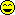Sound pressure, sound or particle velocity, particle displacement or displacement amplitude, (voltage, current, electric resistance). Inverse Distance Law 1/r Sound energy quantities Sound intensity, sound energy density, sound energy, acoustic  power. (electrical power). Inverse Square Law 1/r²

 Note: A sound field quantity (sound pressure p, electric voltage V) is not a sound energy quantity (sound intensity I, sound power Pak). I ~ p2 or P ~ V2. Sometimes you can hear the statement: The cutoff frequency is there where the level L is decreased by (−)3 dB. Whatever the user wants to tell us so accurately: Level is level or dB is dB.